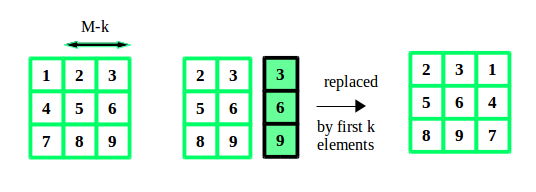# Javascript Program for Rotate the matrix right by K times

• Last Updated : 08 Jun, 2022

Given a matrix of size N*M, and a number K. We have to rotate the matrix K times to the right side.
Examples:

```Input :  N = 3, M = 3, K = 2
12 23 34
45 56 67
78 89 91

Output : 23 34 12
56 67 45
89 91 78

Input :  N = 2, M = 2, K = 2
1 2
3 4

Output : 1 2
3 4```

A simple yet effective approach is to consider each row of the matrix as an array and perform an array rotation. This can be done by copying the elements from K to end of array to starting of array using temporary array. And then the remaining elements from start to K-1 to end of the array.
Lets take an example:## Javascript

 ``

Output:

```23 34 12
56 67 45
89 91 78```

Time Complexity: O(N*M)
Auxiliary Space: O(M)

Please refer complete article on Rotate the matrix right by K times for more details!

My Personal Notes arrow_drop_up